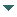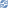# gvSIG

##### Personal tools
You are here: Home Geometry creation
gvSIG DesktopgvSIG Desktop

Cached time 11/21/13 11:24:39# Geometry creation

Up one level
 ◄ Previous Geometry register Next: Geometry examples ►

This document explains how to create a new geometry

The GeometryManager has a method called create with two parameters (type and subtype) which is the generic method to create a new geometry. Using this method, you can create all the geometries previously registered. For example, to create a 2-dimensional point, you must execute the following code:

```GeometryManager geometryManager = GeometryLocator.getGeometryManager();
Geometry point = geometryManager.create(TYPES.POINT, SUBTYPES.GEOM2D);
```

The geometry returned by this method is an empty geometry. To assign a value to the geometry coordinates we must do a casting to the geometry type that we are trying to create. Next, we can fix the coordinate values using the methods that are available in the geometry type. In the next example, we will create a 2-dimensional point in the 2,4 coordinates:

```Point point = (Point) geometryManager.create(TYPES.POINT, SUBTYPES.GEOM2D);
point.setX(2);
point.setY(4);
```

In the next sections of this document, we will comment separately the different geometry types that exist in gvSIG and, for each one, we will show examples of their creation.

 ◄ Previous Geometry register Next: Geometry examples ►